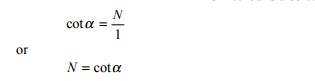Home | | Railways and Airports and Harbour Engineering | Number and Angle of Railway Crossing

# Number and Angle of Railway Crossing

A crossing is designated either by the angle the gauge faces make with each other or, more commonly, by the number of the crossing, represented by N.

Number and Angle of Crossing

A crossing is designated either by the angle the gauge faces make with each other or, more commonly, by the number of the crossing, represented by N. There are three methods of measuring the number of a crossing, and the value of N also depends upon the method adopted. All these methods are illustrated in Fig. 14.10.

Centre line method

This method is used in Britain and the USA. In this method, N is measured along the centre line of the crossing.

Fig. 14.10   Different methods of measuring number (N) and angle of crossingRight angle method

This method is used on Indian Railways. In this method, N is measured along the base of a right-angled triangle. This method is also called Coles method.Isosceles triangle method

In this method, N is taken as one of the equal sides of an isosceles triangle.The right angle method used by Indian Railways, in which N is the cotangent of the angle formed by two gauge faces, gives the smallest angle for the same value of N.

To determine the number of a crossing (N) on site, the point where the offset gauge face of the turnout track is 1 m is marked. The distance of this point (in metres) from the theoretical nose of crossing gives N.

Study Material, Lecturing Notes, Assignment, Reference, Wiki description explanation, brief detail
Civil : Railway Airport Harbour Engineering : Railway Engineering : Points and Crossings : Number and Angle of Railway Crossing |